# 求1~n的连加之和求n的连加之和，有以下思路

## 1，常规思路

``````int sum = 0;
int i = 1;
for(i = 1;i <= n;i++)
sum += i;
return sum;``````

## 2，公式法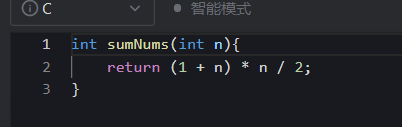``````int sumNums(int n){
return (1 + n) * n / 2;
}``````

使用前n项和的求和公式 n * (n + 1) / 2;

## 3,递归方法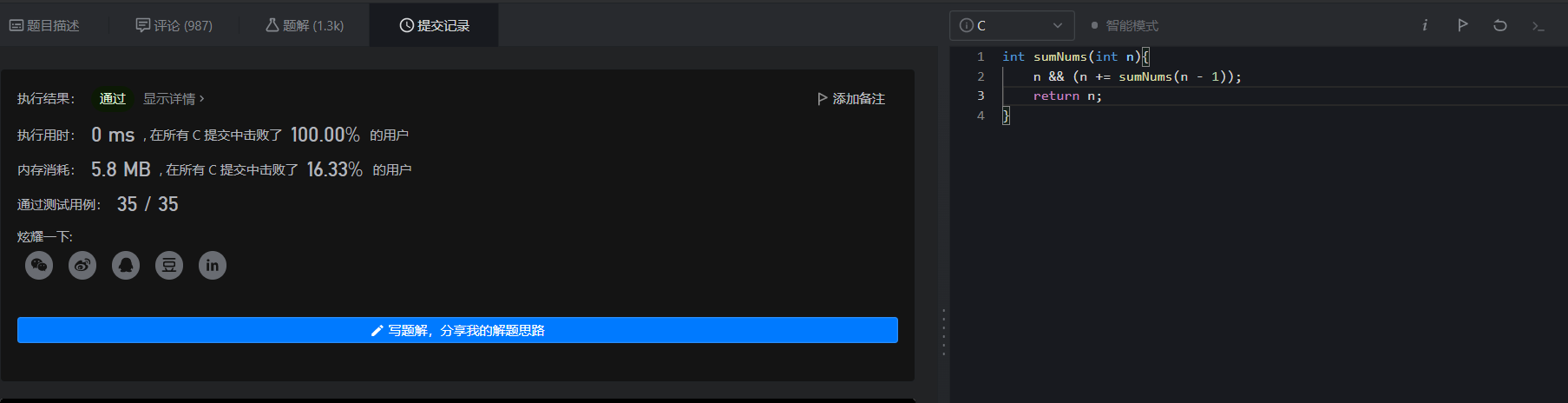``````int sumNums(int n){
n && (n += sumNums(n - 1));
return n;
}``````

# 231. 2 的幂 - 力扣（LeetCode） (leetcode-cn.com)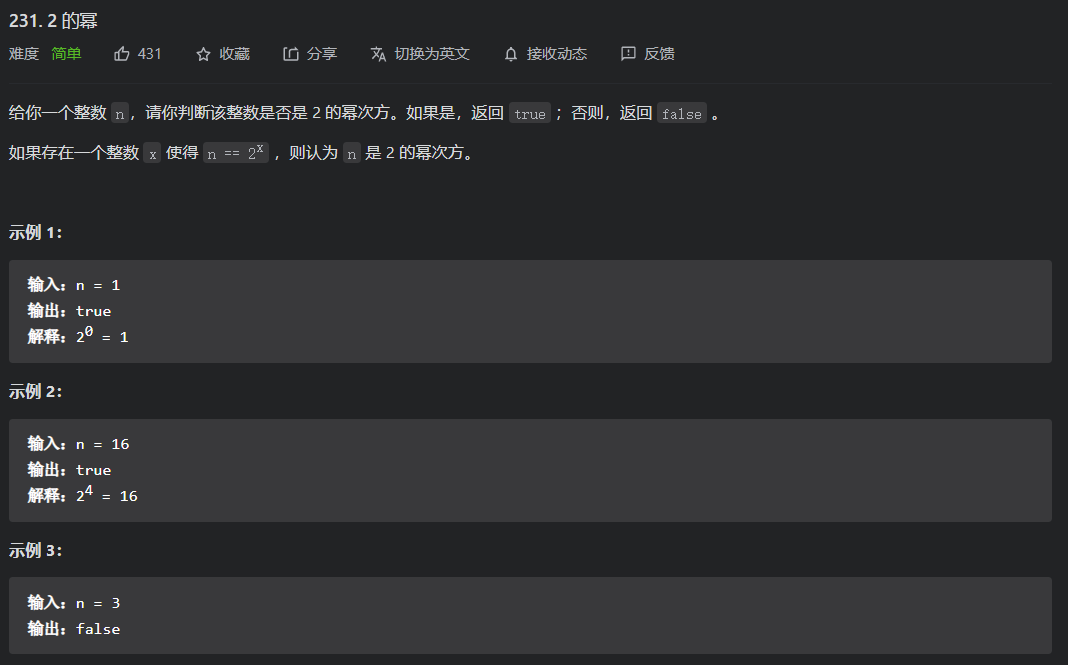## 方法一，穷举法

``````bool isPowerOfTwo(int n){
int i;
unsigned int k = 1;
if(n < 0)
return false;
if(n == 1)
return true;
for(i = 1;i <= 31;i++)
{
k *= 2;
if(k == n)
return true;
}
return false;
}``````

## 方法二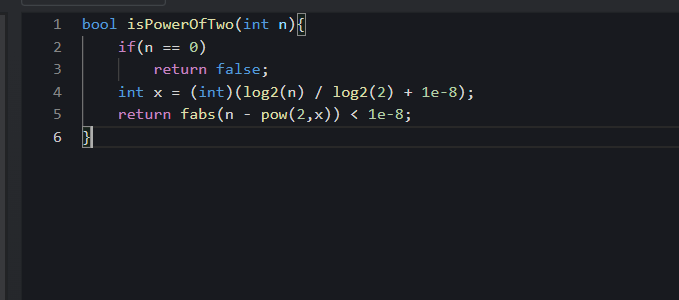``````bool isPowerOfTwo(int n){
if(n == 0)
return false;
int x = (int)(log2(n) / log2(2) + 1e-8);
return fabs(n - pow(2,x)) < 1e-8;
}``````

将原式进行对数运算，得到如上结果，进行浮点数判断

## 方法三，二进制表示

（1），n & （n - 1）== 0

（2），n & ( -n ) == n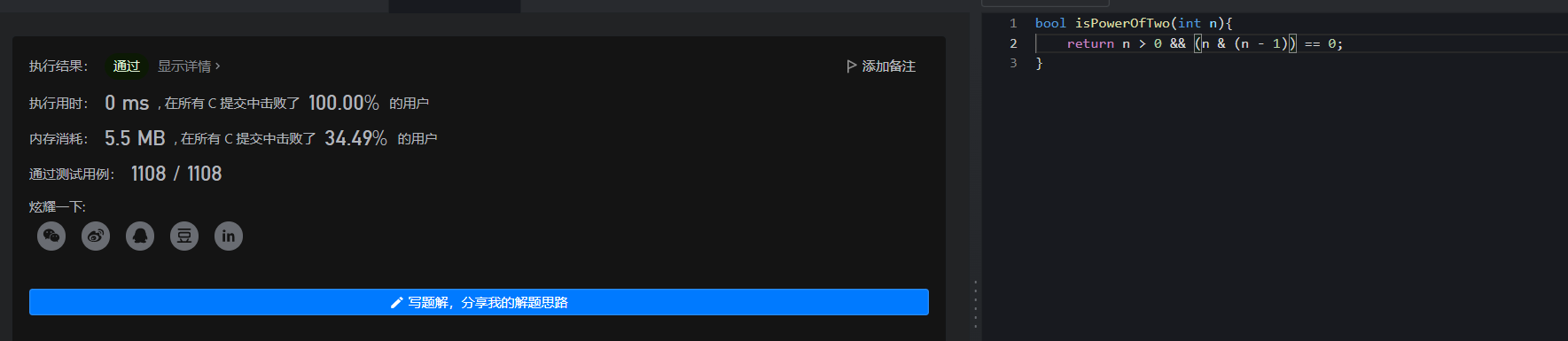``````bool isPowerOfTwo(int n){
return n > 0 && (n & (n - 1)) == 0;
}``````

# 231. 2 的幂 - 力扣（LeetCode） (leetcode-cn.com)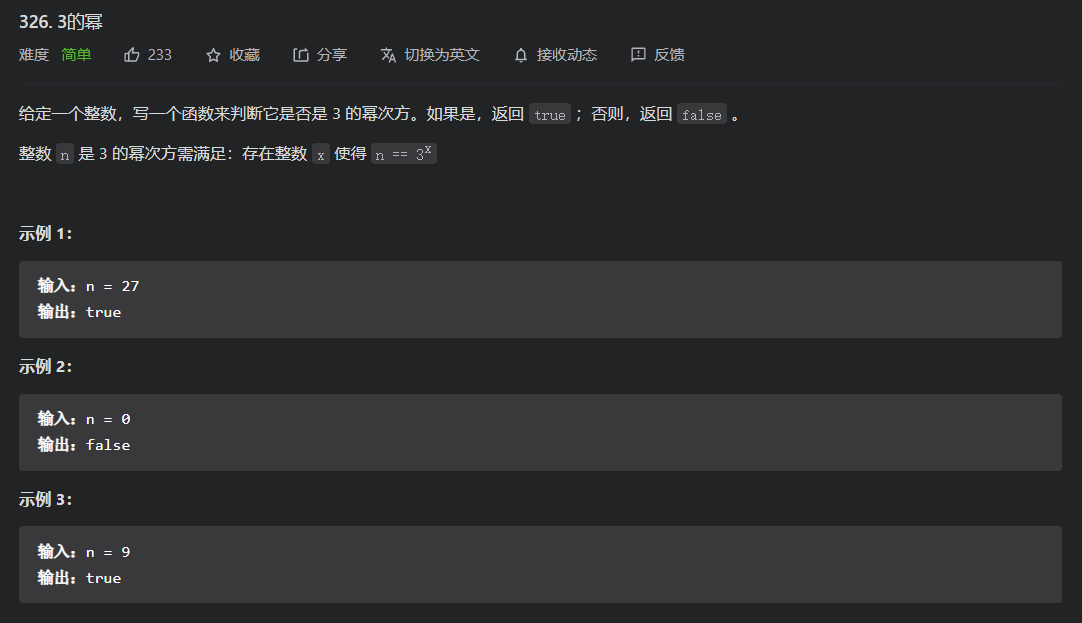# 方法一

## 方法二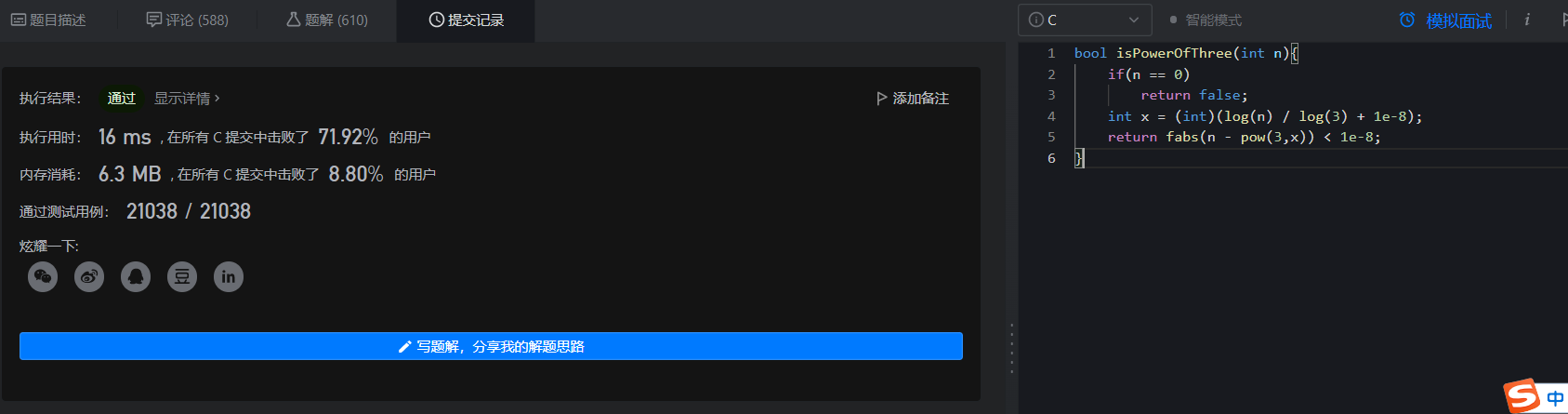对原式进行数学运算，然后进行浮点数精度判断

``````bool isPowerOfThree(int n){
if(n == 0)
return false;
int x = (int)(log(n) / log(3) + 1e-8);
return fabs(n - pow(3,x)) < 1e-8;
}``````

## 342. 4的幂 - 力扣（LeetCode） (leetcode-cn.com)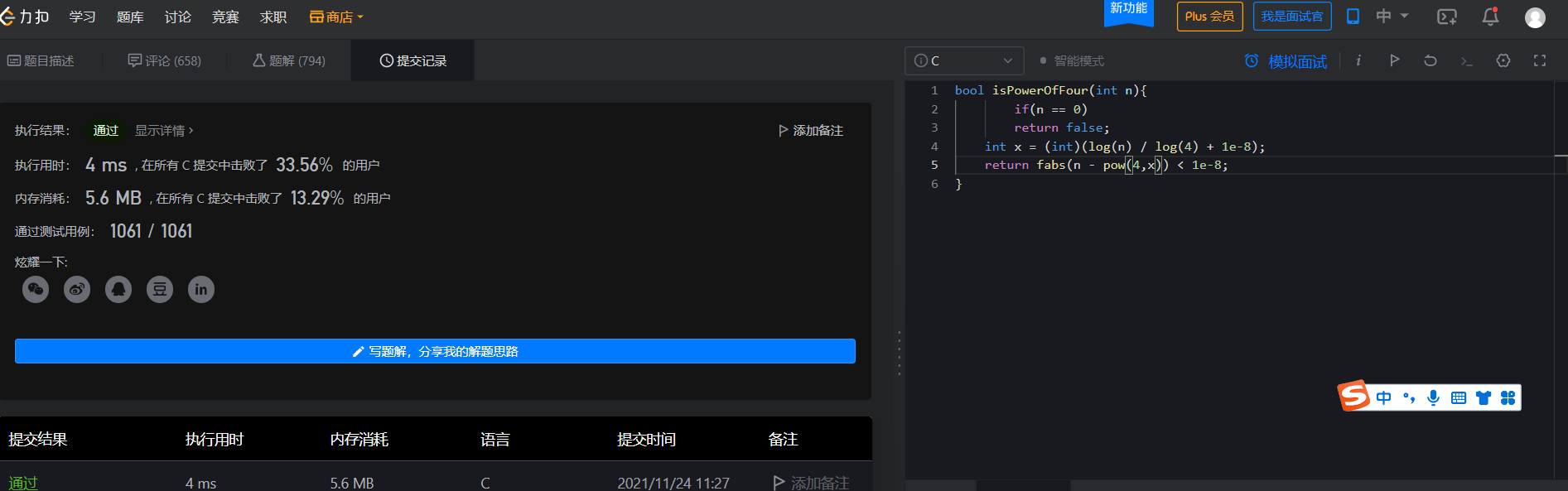# ​​​​​​1492. n 的第 k 个因子 - 力扣（LeetCode） (leetcode-cn.com)## 方法一，枚举法

``````int kthFactor(int n, int k){

int i = 0;
for(i = 1;i <= n;i++)
{
if(n % i == 0)
k--;
if(k == 0)
return i;
}
return -1;
}``````

# 367. 有效的完全平方数 - 力扣（LeetCode） (leetcode-cn.com)## 方法一，使用函数进行判断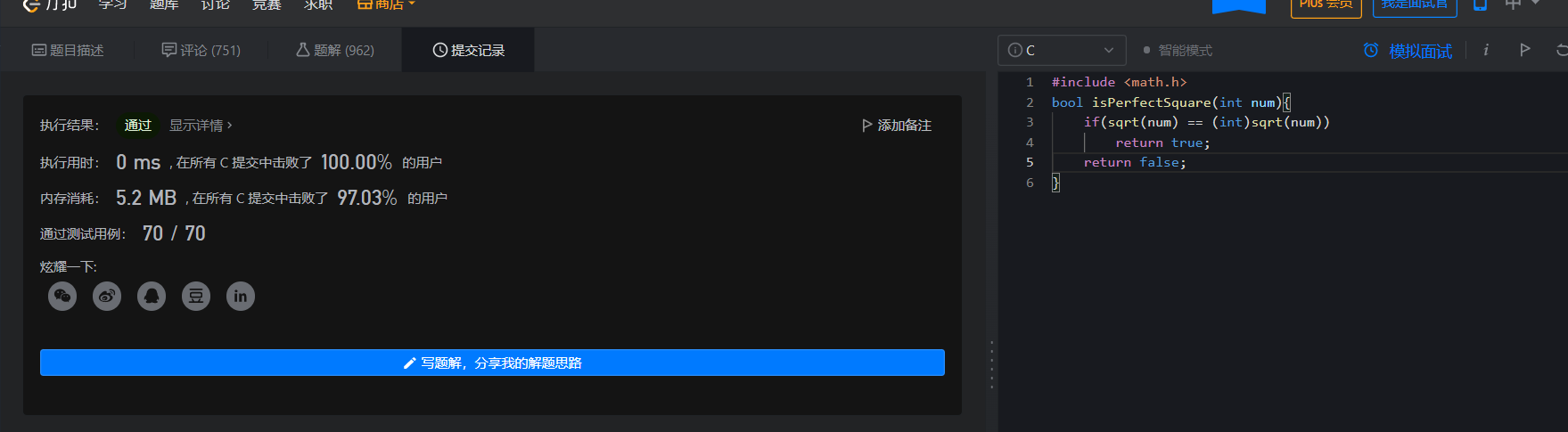``````#include <math.h>
bool isPerfectSquare(int num){
if(sqrt(num) == (int)sqrt(num))
return true;
return false;
}``````

## 方法二：暴力枚举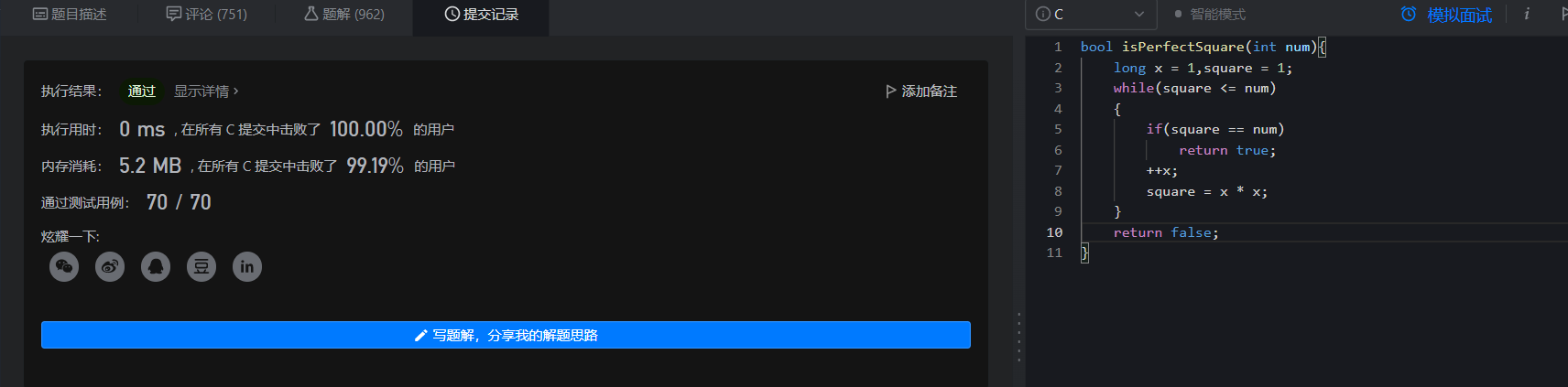``````bool isPerfectSquare(int num){
long x = 1,square = 1;
while(square <= num)
{
if(square == num)
return true;
++x;
square = x * x;
}
return false;
}``````